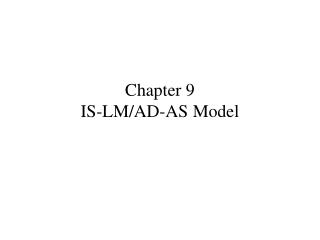DownloadDownload PresentationTélécharger la présentation- - - - - - - - - - - - - - - - - - - - - - - - - - - E N D - - - - - - - - - - - - - - - - - - - - - - - - - - -
##### Presentation Transcript

2. Two Views • Keynesian tradition asserts that government policy can help stabilize the economy • Monetarist and rational expectations tradition: government intervention tends to destabilize the economy. In their view, the market system is self-adjusting

3. II. Income expenditure model . Y = ƒ(capacity utilization) B. Capacity Utilization = ƒ(entrepreneurial expectations regarding receipts) • Productive capacity used depends on entrepreneurial expectations. That is, utilized productive capacity depends on entrepreneurs' expectation of the demand for output

4. C. Expected receipts = ƒ(AD) D. Hence, Keynes’ theory of output and employment is a theory of AD

5. Aggregate demand • AD = C + I + G + (X-IM) • AD= C + I + G +(X-M) • C = Co + cy(Y- To –tY) – crr • I = Io - irr

6. Equilibrium • Equilibrium conditions: • Y = AD • I+ G + X = S + T + IM

7. Y= C+I+G+(X-IM) • Y = Co + cy(Y- To –tY) – crr + Io – irr + G + (X-IM) • Y(1-c+ct) = To + Io + G + (X-IM) – r(cr + ir) • Y= [1/(1-c+ct)]*[To + Io + G + (X-IM) – r(cr + ir)]

8. Multiplier • 1. The multiplier is the multiple by which income changes owing to an autonomous change in expenditures. • 2. Mathematically, the general multiplier is 1/(1-c+ct)

9. 3. The significance of the multiplier is this: small changes in expenditures may result in large changes in output. • Q = Autonomous expenditures * Multiplier • Gov’t multiplier • tax multiplier • Balanced budget multiplier

10. IS-LM Analysis • IS-LM analysis represents an interpretation of Keynes' General Theory stemming from J.R. Hick's class article entitle "Keynes and the Classics." Hicks argues that the essence of Keynes' theory is his theory of liquidity preference. Individuals hold money (liquidity) for transactions, for speculative reasons, and for emergencies.

11. IS-LM analysis allows us to solve for income and the interest rate simultaneously. Hence, IS-LM is a general equilibrium system. In this regard, IS-LM analysis is a powerful tool enabling us to analyze the impacts of changes in policy on the economy.

12. a dichotomy between the goods market and the money markets and • equilibrium in both markets.

13. FE Line: Equilibrium in the Labor Market • Full employment line corresponds to that level of output at which labor is fully employed • Shifts in the FE line: • Increase in the labor supply • Change in the capital stock • Change in technology

14. IS curve • Equilibrium in the goods market • Expresses an inverse relation between interest rates and income, assuming equilibrium in the goods market (I=S, or AD=Y)

15. Shifts in the IS curve: • ↑ C, I, G, X →↑IS • ↑ S, T, M →↓IS

16. LM curve: • Equilibrium in the money market or financial assets market • Expresses a positive relationship between interest rates and income, assuming equilibrium in the money (asset) market (Md=Ms)

17. Shifts in the LM curve: • ↑ Ms →↑ LM • ↑ Mt, Msp, P →↓ LM • Where Ms = money supply • Mt = transactions demand for money • Msp = speculative or asset demand for money

18. A Temporary Adverse Supply Shock • Assume that bad weather or increases in oil prices reduce productivity • Impact: Production function shifts down, causing the demand for labor to decline • Full employment output contracts • Result: IS LM intersect to the right of full employment output • Price level increase • This reduces the real money supply (Ms/P) leading to an decrease in LM

19. Effect of a monetary expansion

20. Monetarists Assume Investment is sensitive to interest rates Ignore the speculative motive Fiscal Policy is ineffective (crowds out private investment) Monetary policy is very effective Keynesians Assume Investment is insensitive to interest rates (expectations are more important) Md = Mt+Msp Fiscal Policy is effective Monetary policy less effective, ineffective in the liquidity trap Differences between Keynes and the monetarists

21. Self adjusting economy • If IS LM intersect to the right of full employment, price level increases shifting LM left • If IS LM intersect to the left of full employment, price level decreases shifting LM right • Even in the liquidity trap, decline in the price level will increase money denominated wealth, thereby increasing consumption • Classical: price level adjusts rapidly • Keynesian: price level adjusts slowly

22. Aggregate Demand and Aggregate Supply Model • Aggregate Demand curve: • Expresses an inverse relation between price level and expenditures • Shifts in the AD curve

23. Aggregate Supply Curve • 3 versions • Keynesian AS: P is constant • Short run AS: Y = Yfe + b(P-Pe) • Long run AS: Y = Yfe(P=Pe) • Shifts in AS • Any factor that shifts level of Yfe shifts the LR AS curve • Shifts in the Keynesian AS: caused by changes in Prices that firms charge

24. Neutrality of Money • Money is neutral in the long run • Money is not neutral in the short run